# Binary Division (How To Guide With Rules And Examples)

## How to Divide Binary Numbers

Binary division is an important but often overlooked part of binary arithmetic.

Binary addition, binary subtraction, binary multiplication, and binary division are the four types of arithmetic operations that occur in binary arithmetic.

Although binary division is not too difficult, it can initially be a bit harder to understand than the other binary operations.

This is because all the other binary operations share similarities with each other, whereas binary division is a bit of an outlier.

For example, all of the other binary operations had four basic steps which made them a bit easier to understand.

But the process of binary division does not have any specific rules to follow—although it is quite similar to decimal division.

To make this a bit clearer, let’s look at a binary division example problem.

Let us take A = 11010 and B = 101, where we want to divide A by B.

The structure of the operation of binary division is similar to that of decimal division, now we will look into the operation step by step to make it understanding as much as possible.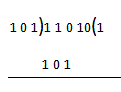In the first step, the left-most digits of dividend i.e. A are considered, and depending upon the value the divisor is multiplied with 1 and the result which is the result of multiplication of 101 and 1 are written.

As we already know that 1 × 1 = 1, 1 × 0 = 0 and 1 × 1 = 1. we get: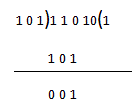In this step 101 is subtracted from 110 (see the binary subtraction for help with that)

Now going into the next step: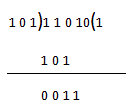As per the rules of division, the next least significant bit comes down and we try to multiply 1 with divider i.e. B but the result is bigger than the minuend so this step cannot be completed and we have to go to the next step.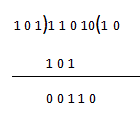0 is inserted into the quotient and the least significant bit comes down now we can proceed to the next step.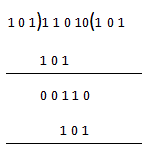Now again the divisor is multiplied by 1 and the result is written, the result is similar to the first one because all the numbers are the same.

Now we are going into the final step.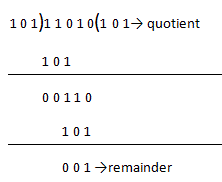In the final step, binary subtraction is done and we get the remainder and the operation of binary division is completed and we get the following result.

Quotient = 101 and remainder = 1.

All done!